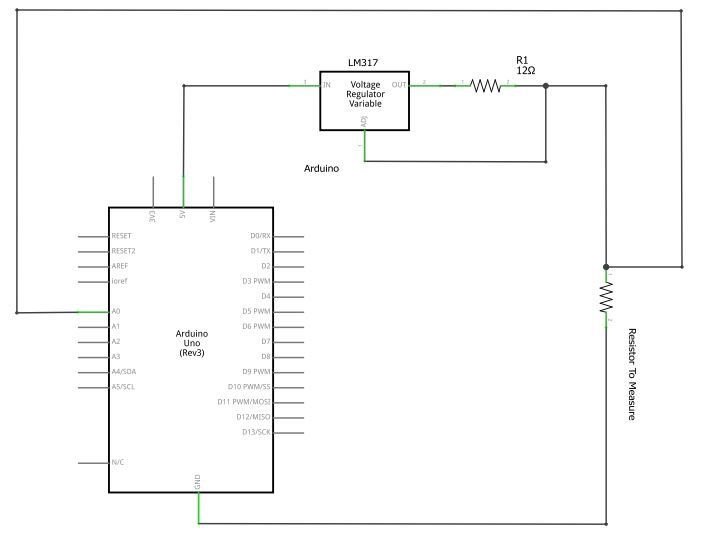# Reading Milliohm Resistances With The Arduino

I’ve recently been attempting to read milliohm resistance with the Arduino, and I’ve found out it isn’t terribly easy. The main cause is that the Atmega chips 10bit ADC doesn’t provide the resolution needed. As a result, I’ve been hunting for a different method, and I have come up with what I think is the best solution.

Using four-terminal sensing, or kelvin resistance measurements, we will use a constant current supply and some Ohm’s Law-Fu to read a voltage that is proportional to the resistance.

Lets start with some theory, and Ohm’s Law. First off, we are going to be looking for resistance, so lets put Ohm’s Law in terms or resistance:$r= frac{v}{i}$

Now we know that we need voltage and current to determine the resistance. So, we’ll need to build a current supply, with a constant output. For example, if we generate a constant 1 amp current, than we will have a handy relationship:$r= frac{v}{1} = v$

Given a 1 amp source, we are given that resistance is 1:1 proportional to the voltage.

Lets take a look at a circuit.This circuit is our constant current supply. Using the LM-317, and a resistor between the adjust pin, and the voltage out pin, we create a basic constant current supply. Using a 120 ohm resistor, we will be generating a 1amp current. However, when we use Arduino, we have the power to do some calculations, we we are going to scale the current down by a factor of 10, and replace the 120 ohm resistor with 12 ohm resistor, resulting in a current of 100mA, or 0.1amps.

With our constant current supply, the next step is to measure the voltage across the resistor we want to measure.Now we have our constant current supply powered by the Arduino, supplying 100mA across our resistor. We are going to run from the resistor, to the A0 pin on the Arduino, and the other side to ground. Any low ohm resistor can be put in place of the 12ohm resistor, just keep in mind that you’ll have the read the resulting current with a multimeter, than enter that value into the code. In my example, I built with a 10ohm resistor, that resulted in ~125mA, so my code uses 0.125 as my current.

Lets go ahead and throw some code onto the Arduino, and try to read the voltage, do some math to generate the resistance, and read it out!

``````const float currentSupply = 0.125; // The current generated by the LM317 and 10ohm Resistor
const float referenceVolts = 5;        // the default reference on a 5-volt board
const float resistorFactor = 1023; //Full scale this time.
const int resistancePin = 0;         //Resistor / output from 317 circuit connected to analog pin 0
void setup()
{
Serial.begin(9600);
}

void loop()
{